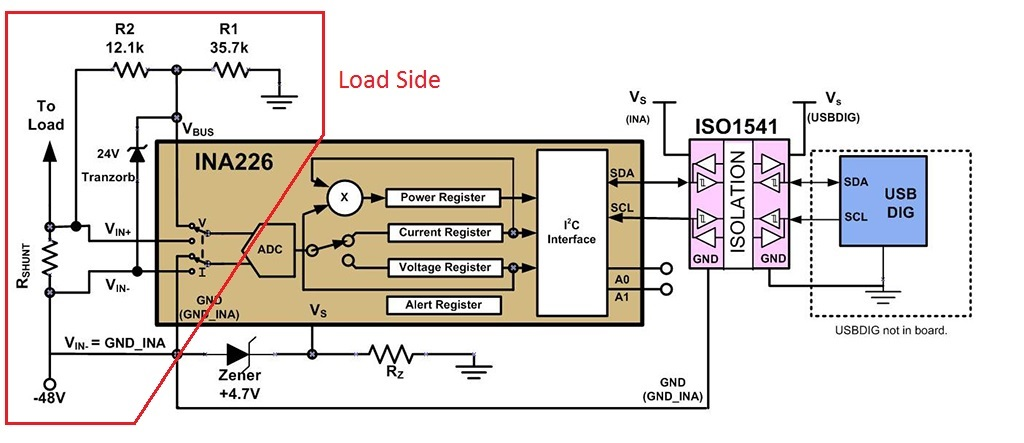If you have a related question, please click the "Ask a related question" button in the top right corner. The newly created question will be automatically linked to this question.

# TIDA-00313: TIDA-00313 GND_ISO current going through shunt resistor error

Part Number: TIDA-00313
Other Parts Discussed in Thread: INA226

After studying the TIDA-00313 schematic and filling in the blanks of how a -48V supply would be connected, it appears to me that the VBUS voltage divider current is going through the shunt resistor and causing a measurement error that could be eliminated if R2 (12.1K) was connected to VIN- instead of VIN+.

Can you confirm?

Thanks

• Hello Frank,

It depends on what current you are trying to measure. If you want the current of the whole system, then you would want R2 connected to VIN+, If you want the current of the Load only, then you would want it connected to VIN-. Note that if R2 is connected to VIN- then there will be a relatively small error introduced in the voltage measurement.
• I have some questions regarding your response:
1) The whole system current is would not be measured by connecting R2 to VIN+ since the current consumed via the VS pathway is not taken into account.
2) Can you explain why there would be a error voltage introduced by connecting R2 to VIN-? (I don't see it and would like if you could explain it)
3) I'd like to verify that I'm looking at the -48V supply and load connections correctly and the same as you are.
The way I looking at it is:
J1-3 (GND_ISO) gets directly connected to the positive terminal of the power supply (Or battery)
J1-2 (VIN-) gets gets directly connected to the negative terminal of a power supply (Or battery)
J1-1 (VIN+) gets connected to one side of the load
The other side of the load gets connected to (GND_ISO)

Is that correct?

Thanks
• Hello Frank,

For your first question, I meant the whole system on the Load side. Sorry, I wasn't very clear.

For your second question, there is a small error in the voltage measurement because if R2 is connected to VIN- then you are looking at the Bus Voltage of the load and the shunt, as opposed to just the load. However, this voltage difference is very small.

Third, I believe you are looking at the supply and load connections correctly.
• Hi Mitch,
If we are looking at the same supply and load connections, then I'm sorry but I don't understand your answers at all.
What do you mean by the "whole system on the load side" (I just can't follow what you are talking about, a picture would really help)
The voltage measurement is taken as the difference between VIN+ & VIN- correct? (So what do you mean by the "bus voltage of the load"
If you actually drew the connections as I explained them and referenced the 3 terminals of J1 and the load connections as I explained them, and if the voltage measurement is taken as the difference between VIN+ and VIN- , then what you are saying makes no sense to me.

Please confirm that you have actually drawn in the connections like I stated and analyzed the resulting circuit. (I'm thinking we are still looking at different power supply and load connections)

Respectfully,
Frank
• Hello Frank,

Here is an image of what I meant by the load side:The voltage measurement that I was referencing was not the differential voltage across VIN+ and VIN-, but Vbus voltage measurement. From the diagram above you can see that the INA226 takes two different measurements. One measurement is for the current (the differential voltage), and the other measurement is for the bus voltage Vbus.  This second measurement allows the INA226 to calculate the power.  So, to clarify, in the above picture, the INA226 is measuring the bus voltage with the Vbus pin on the Load side of Rshunt.  If you were to connect R2 to Vin- then Vbus would be measured at the -48V side of Rshunt, which will be slightly different.

• Thanks, now I understand, but unfortunately you either get a more accurate VBUS measurement (with R2 connected to VIN+) assuming that the divider is taken into account, or a more accurate current measure with R2 connected to VIN-. I would go with the more accurate current measurement since the divider is already introducing a measurement error. Do you agree?
• Figure 7 in the TIDU361.pdf document proves my assertion that R2 should be connected to VIN-

With R2 connected to VIN+ the VBUS voltage measurement gets corrupted by a load current dependent error as the voltage drop across the shunt resistor gets added to the VBUS voltage measurement.

The schematic Figure A-1 is simply wrong and should be acknowledged and corrected.

QED

• Correct, Figure 7 shows that it is fine to connect R2 to VIN-.

• Paragraph 2.1.2 also states that
• Paragraph 2.1.1 also states "Please note that INA226 measures the bus voltage, Vbus, with respect to
GND_INA." Figure 6 further illustrates R2 connected to VIN-.
Therefore it is absolutely wrong to connect R2 to VIN+.
It will produce a load current dependent error and a constant current error offset since the divider current is erroneously going through R shunt.
I think the measurement data also reflects this error as the graphs show a non-linear behavior.
The eval board clearly has an error and it should be acknowledged by TI as such and move on.
Cheers